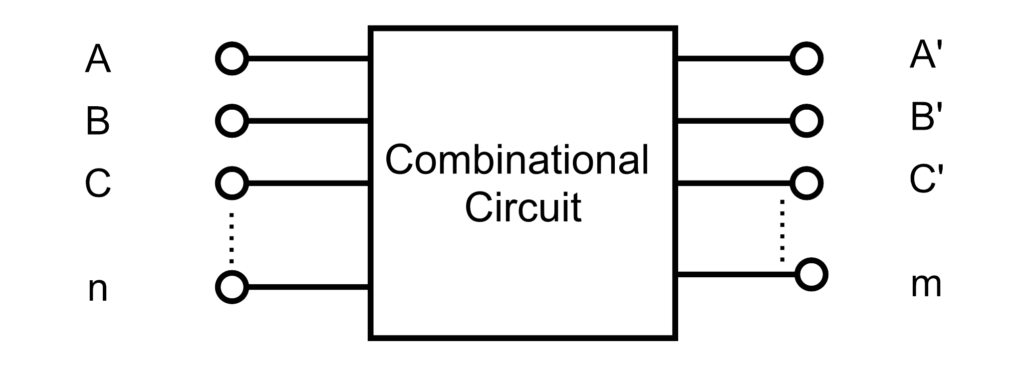### Difference Between Combinational Circuits & Sequential Circuit in Tabular Form.

 SNO. Combinational Circuits Sequential Circuit 1. DiagramCombinational Circuits DiagramSequential Circuit 2. Output depends only on the past values of input. Output depends on the present and past values of input form Memory. 3. Feedback path is not used in Combinational Circuits. Feedback path is used for sequential circuit. 4. Memory element is not present. Memory element is present. 5. Clock is not used in Combinational circuits. Clock is used in sequential circuits. 6. Circuit is simple. Circuit is complex. 7. Examples of combinational circuits are Adders subtractors code converters comparators multuplexer, de-multiplexer, decoder, encoder, etc. Examples of sequential circuits are : Flip-flops, counters, registers etc.

(Visited 137 times, 1 visits today)

Written by:

### One thought on “Combinational Circuits & Sequential Circuit”

• 20/06/2018 at 11:31 am